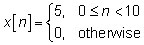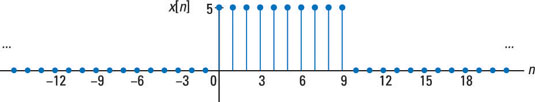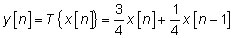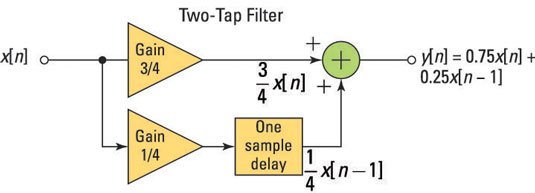##### Signals and Systems For DummiesDiscrete-time signals and systems march along to the tick of a clock. Mathematical modeling of discrete-time signals and systems shows that activity occurs with whole number (integer) spacing, but signals in the real world operate according to periods of time, or the update rate also known as the sampling rate.

Discrete-time signals, which can also be viewed as sequences, only exist at the ticks, and the systems that process these signals are, mathematically speaking, resting in the periods between signal activity.

Systems take inputs and produce outputs with the same clock tick, generally speaking. Depending on the nature of the digital hardware and the complexity of the system, calculations performed by the system continue — between clock ticks — to ensure that the next system output is available at the next tick when a new signal sample arrives at the input.

## Discrete-time signals

Discrete-time signals are a function of time index n. Discrete-time signal x[n], unlike continuous-time signal x(t), takes on values only at integer number values of the independent variable n. This means that the signal is active only at specific periods of time. Discrete-time signals can be stored in computer memory because the number of signal values that need to be stored to represent a finite time interval is finite.

The following simple signal, a pulse sequence, is shown as a stem plot — a plot where you place vertical lines, starting at 0 to the sample value, along with a marker such as a filled circle. The stem plot is also known as a lollipop plot — seriously.The stem plot shows only the discrete values of the sequence.

## Discrete-time systems

A discrete-time system, like its continuous-time counterpart, is a function, T{}, that maps the input x[n] to the output y[n] = Y{x[n]}. An example of a discrete-time system is the two-tap filter:The term tap denotes that output at time instant n is formed from two time instants of the input, n and n – 1. Check out a block diagram of a two-tap filter system.In words, this system scales the present input by 3/4 and adds it to the past value of the input scaled by 1/4. The notion of the past input comes about because x[n – 1] is lagging one sample value behind x[n]. The term filter describes the output as an averaging of the present input and the previous input. Averaging is a form of filtering.